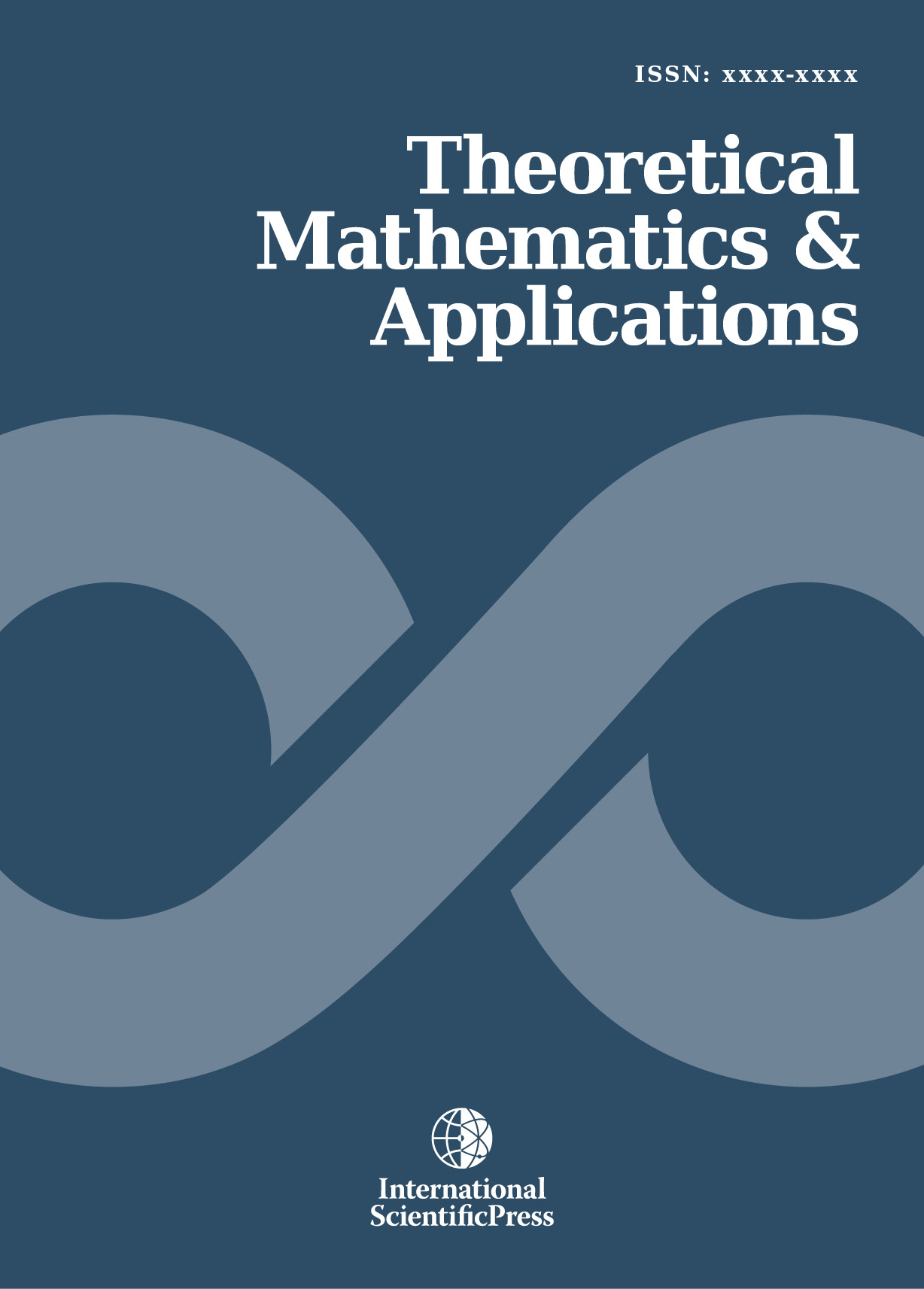# Theoretical Mathematics & Applications

#### A Numerical Method for Prediction of Masses of Real Particles, e.g. Neutrinos

•[ Download ]
• Times downloaded: 507
• Abstract

It is shown how particle quantities like masses of supposed neutrinos can be computed on base of a classical theory. The numerical method is based on discretization of differential equations which is being used successfully in some parts of theoretical physics but is underestimated wrongly for application to particles. The method is explained by an ordinary differential equation first. Then it is demonstrated how this simple method proves successful for non-linear field equations with chaotic behaviour. Integration constants of the field equations enter the method in form of parameters. Using certain discrete values of the integration constants, a chaos-like behaviour comparable with Mandelbrot sets is obtained. The known Einstein-Maxwell equations are investigated, where discrete particle quantities are obtained from a continuous theory which is possible only by this method. Known particle values are confirmed, and unknown values can be predicted. In this paper, supposed neutrino masses are presented.ISSN: 1792-9687 (Print)
1792-9709 (Online)